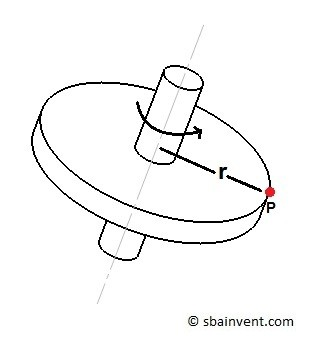# Force & Acceleration on a Rigid Body

A body that is experiencing acceleration or deceleration will be subjected to a force.  This is due to Newton’s second law of motion which states the following.

“The relation between an object’s mass m, its acceleration a, and the applied force F is F=ma, where acceleration and force are vectors, and the direction of the force vector is the in the same direction as the acceleration vector.”

In other words the force of a body is equal to its mass times acceleration.

#### Forms of Motion on a Rigid Body

In the previous section “Kinematics of a Rigid Body”  I discuss how a rigid body will behave as it experience motion.  There are three types of motion that a rigid body could experience.

###### Translation

One type of motion would be translation.  Translation is very straight forward.  As a rigid body moves an observer will be able see the paths of all the particle within the body move in parallel to each other.  Due to this fact the force on each particle will be the same, and the same principle used to calculate the forces on a particle can be use.###### Rotation about a Fixed Axis

The next type of motion that a rigid body could experience is rotation about a fixed axis.  Recall that as a body rotates it will always have a normal acceleration even if the velocity is constant.  There will always be a normal force that become greater as you move away from the axis of rotation.  It can also have an angular acceleration if its rotational velocity changes.As the body rotates it will develop a mass moment of inertia.  The mass moment of inertia is the resistance of a body to angular acceleration. Mass moment of inertia is dependent on the body’s mass and the distance the mass is from the axis of rotation.

###### General Plane Motion

Finally,  a rigid body can also experience a combination of translation and rotation about a fixed axis.  For example the coupling link in a linkage will experience general plane motion.  General plane motion can cause the body to experience different forces in different directions at different point in the body.  Due to this, the analysis for general plane motion can become complex, and different methods of analysis such as absolution motion, and relative motion analysis will need to be used.

Next

|